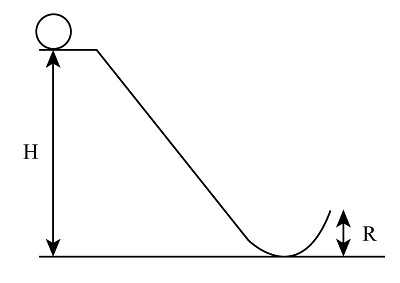# A small circular object with mass m and radius r has a moment of inertia given by I = cmr^2. The...

## Question:

A small circular object with mass m and radius r has a moment of inertia given by I = cmr{eq}^2. {/eq} The object rolls without slipping along the track shown in the figure. The track ends with a ramp of height R = 3.0 m that launches the object vertically. The object starts from a height H = 9.0 m. To what maximum height will it rise after leaving the ramp if c = 0.39?

## Angular Velocity:

When the object is in angular motion, then the speed of the object changes for a particular given time, this change in the speed of the object for a particular period of time is known as the angular velocity of the object.

Given data:

• Mass of circular object is {eq}m {/eq}
• Radius of circular object is {eq}r {/eq}
• Moment of inertia, {eq}I = cm{r^2} {/eq}
• Height at end, {eq}R = 3.0\;{\rm{m}} {/eq}
• Height at initial point, {eq}H = 9.0\;{\rm{m}} {/eq}
• And, {eq}c = 0.39 {/eq}By applying the law of conservation of energy:

{eq}mgH = mgR + \dfrac{1}{2}m{v^2} + \dfrac{1}{2}I{\omega ^2} {/eq}

Here, linear velocity is {eq}v = r\omega {/eq}

{eq}\begin{align*} mgH &= mgR + \dfrac{1}{2}m{v^2} + \dfrac{1}{2}cm{r^2}{\left( {\dfrac{v}{r}} \right)^2}\\ mgH &= mgR + \dfrac{1}{2}m{v^2} + \dfrac{1}{2}cm{v^2}\\ {v^2} &= 2g\left( {H - R} \right)\left( {1 + c} \right) \end{align*} {/eq}

Substitute the given values

{eq}\begin{align*} {v^2} &= 2 \times 9.8\left( {9 - 3} \right)\left( {1 + 0.39} \right)\\ v &= 12.78\;{\rm{m/s}} \end{align*} {/eq}

From the 3rd kinematic equation

{eq}{v^2} - {u^2} = 2g\left( {{R_o} - R} \right) {/eq}

Here, {eq}u = 0 {/eq} initial velocity of object.

{eq}{v^2} = 2g\left( {{R_o} - R} \right) {/eq}

Substitute the given values

{eq}\begin{align*} {\left( {12.78} \right)^2} &= 2 \times 9.8\left( {{R_o} - 3} \right)\\ 163.32 &= 19.6{R_o} - 58.8\\ {R_o} &= 11.3\;{\rm{m}} \end{align*} {/eq}

Therefore, {eq}11.3\;{\rm{m}} {/eq} height it will rise after leaving the ramp.